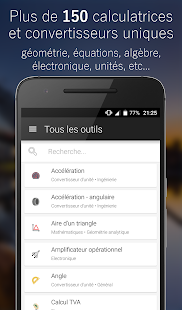CalcKit All in One Calculator Premium 2.2.4 - APK Neo

# CalcKit All in One Calculator Premium 2.2.4

Discription :Great collection of electronic tools, mathematical calculations and unit converters.
All in one calculation package, a must for all students and enthusiasts.
With more than 80 calculators and converters, CalcKit is the only toolbox for calculations needed on your device.
+ The first floating RPN calculator for Android
+ 48 Tools for mathematical calculations
+ 22 Tools for electronics
+ 14 Unit Converter
+ Custom Tool Maker
+ Support for input of mathematical expressions
+ Support for tablet
+ 3 different themes

Screen Shots